## Example Questions

← Previous 1

### Example Question #11 : Equilateral Triangles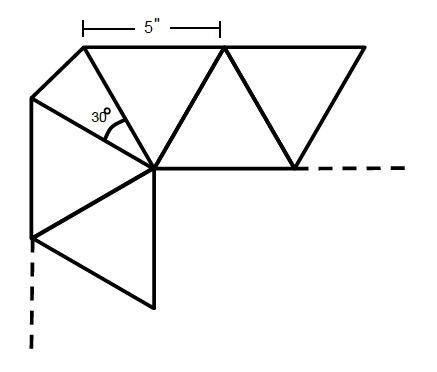A square rug border consists of a continuous pattern of equilateral triangles, with isosceles triangles as corners, one of which is shown above. If the length of each equilateral triangle side is 5 inches, and there are 40 triangles in total, what is the total perimeter of the rug?

The inner angles of the corner triangles is 30°.

124

200

188

208

180

188

Explanation:

There are 2 components to this problem. The first, and easier one, is recognizing how much of the perimeter the equilateral triangles take up—since there are 40 triangles in total, there must be 40 – 4 = 36 of these triangles. By observation, each contributes only 1 side to the overall perimeter, thus we can simply multiply 36(5) = 180" contribution.

The second component is the corner triangles—recognizing that the congruent sides are adjacent to the 5-inch equilateral triangles, and the congruent angles can be found by

180 = 30+2x → x = 75°

We can use ratios to find the unknown side:

75/5 = 30/y → 75y = 150 → y = 2''.

Since there are 4 corners to the square rug, 2(4) = 8'' contribution to the total perimeter. Adding the 2 components, we get 180+8 = 188 inch perimeter.

### Example Question #2 : How To Find The Perimeter Of An Equilateral Triangle

The height of an equilateral triangle is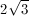What is the triangle's perimeter?

6

12

24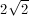8

12

Explanation:

An altitude drawn in an equilateral triangle will form two 30-60-90 triangles. The height of equilateral triangle is the length of the longer leg of the 30-60-90 triangle. The length of the equilateral triangle's side is the length of the hypotenuse of the 30-60-90.

The ratio of the length of the hypotenuse to the length of the longer leg of a 30-60-90 triangle is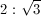The length of the longer leg of the 30-60-90 triangle in this problem isUsing this ratio, we find that the length of this triangle's hypotenuse is 4. Thus the perimeter of the equilateral triangle will be 4 multiplied by 3, which is 12.

### Example Question #1 : How To Find The Height Of A Triangle

A triangle has a base of 5 cm and an area of 15 cm. What is the height of the triangle?

3 cm

1.5 cm

None of the above

6 cm

5 cm

6 cm

Explanation:

The area of a triangle is (1/2)*base*height. We know that the area = 15 cm, and the base is 5 cm, so:

15 = 1/2 * 5 * height

3 = 1/2 * height

6 = height

### Example Question #541 : Psat Mathematics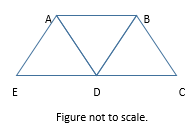In the figure above, AB = AD = AE = BD = BC = CD = DE = 1. What is the distance from A to C?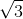Explanation: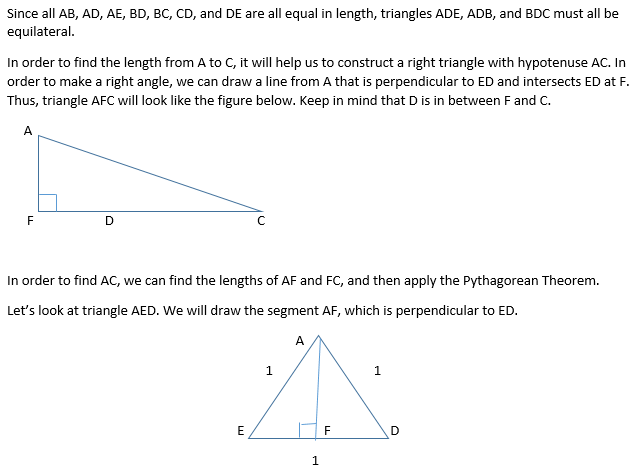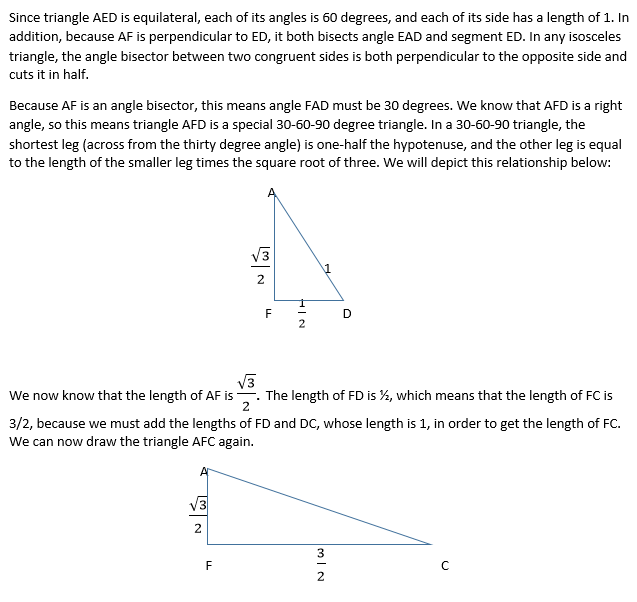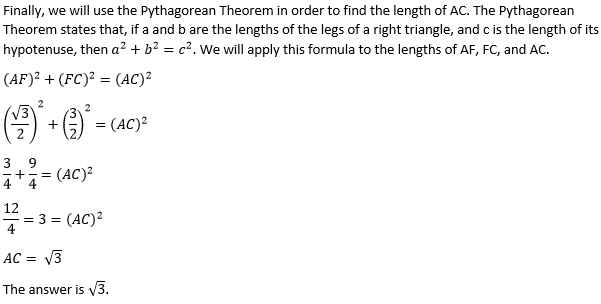### Example Question #3 : How To Find The Height Of A Triangle

A triangles has sides of 5, 9, and. Which of the folowing CANNOT be a possible value of?Explanation:

The sum of the lengths of the shortest sides of a triangle cannot be less than the third side.

3 + 5 = 8 < 9, so 3 can't be a value of x.

### Example Question #1 : Equilateral Triangles

An equilateral triangle has a side length of 4. What is the height of the triangle?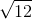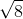Explanation:

Because the triangle is an equilateral triangle, you know that all sides have the same length, so all sides have the length of 4. Draw the triangle and label all sides as 4. Next, draw a point in the middle of one of the sides, and label each side as 2. Draw a line segment from the midpoint that you just created to the opposite angle of the triangle. That line segment is the height of the triangle. You can solve for it by using the Pythagorean theorem.

The Pythagorean theorem states thatIn our scenario a = 2, c = 4, and you are solving for b. After plugging the numbers into the formula you get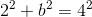and then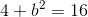and then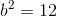and then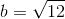Therefore, the height of the triangle is### Example Question #2 : How To Find The Area Of An Equilateral Triangle

The area of square ABCD is 50% greater than the perimeter of the equilateral triangle EFG. If the area of square ABCD is equal to 45, then what is the area of EFG?

50

30

25√3

50√3

25

25√3

Explanation:

If the area of ABCD is equal to 45, then the perimeter of EFG is equal to x * 1.5 = 45. 45 / 1.5 = 30, so the perimeter of EFG is equal to 30. This means that each side is equal to 10.

The height of the equilateral triangle EFG creates two 30-60-90 triangles, each with a hypotenuse of 10 and a short side equal to 5. We know that the long side of 30-60-90 triangle (here the height of EFG) is equal to √3 times the short side, or 5√3.

We then apply the formula for the area of a triangle, which is 1/2 * b * h. We get 1/2 * 10 * 5√3 = 5 * 5√3 = 25√3.

In general, the height of an equilateral triangle is equal to √3 / 2 times a side of the equilateral triangle. The area of an equilateral triangle is equal to 1/2 * √3s/ 2 * s = √3s2/4.

### Example Question #1 : How To Find The Area Of An Equilateral Triangle

What is the area of an equilateral triangle with sides 12 cm?

54√2

12√2

18√3

36√3

72√3

36√3

Explanation:

An equilateral triangle has three congruent sides and results in three congruent angles. This figure results in two special right triangles back to back: 30° – 60° – 90° giving sides of x - x √3 – 2x in general. The height of the triangle is the x √3 side.  So Atriangle = 1/2 bh = 1/2 * 12 * 6√3 = 36√3 cm2.

### Example Question #1 : Equilateral Triangles

A regular hexagon and an equilateral triangle have equal perimeters.  If the hexagon has side lengths of 5, what is the area of the triangle?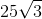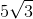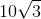Explanation:

Perimeter of hexagon =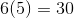= perimeter of triangle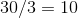= side of the triangle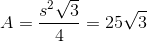### Example Question #13 : Equilateral Triangles

An equilateral triangle has a perimeter of 18. What is its area?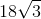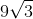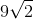Explanation:

Recall that an equilateral triangle also obeys the rules of isosceles triangles.   That means that our triangle can be represented as having a height that bisects both the opposite side and the angle from which the height is "dropped."  For our triangle, this can be represented as: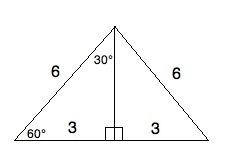Now, although we do not yet know the height, we do know from our 30-60-90 regular triangle that the side opposite the 60° angle is √3 times the length of the side across from the 30° angle. Therefore, we know that the height is 3√3.

Now, the area of a triangle is (1/2)bh. If the height is 3√3 and the base is 6, then the area is (1/2) * 6 * 3√3 = 3 * 3√3 = 9√(3).

← Previous 1

### All SAT Math Resources